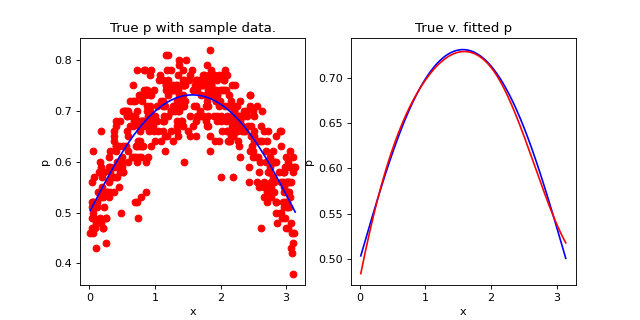# Welcome to binney’s documentation!¶

binney is a package for doing binomial regression (including logistic regression) with b-splines! See below for installation instructions.

## Installation¶

You can install binney with pip:

pip install binney


You will also need to install ipopt, which is an interior point optimizer, with conda.

conda install -c conda-forge cyipopt


You can check to see if your installation worked correctly with pytest.

# pip install pytest
cd binney
pytest


## Documentation¶

For instructions on how to use binney, see the API reference for binney.run.run.BinneyRun.

## Quick Start¶

Here is a quick introduction to simulate data and do a BinneyRun. Please refer to binney.run.run.BinneyRun for documentation on its arguments and methods. We will simulate 500 observations from

$\begin{split}x \sim Uniform(0, \pi) \\ k \sim Binomial(n=100, p=p(x)) \\\end{split}$

where $$p(x) = \frac{e^{sin(x)}}{1 + e^{sin(x)}}$$. First, let’s create the data frame representing this simulation.

import matplotlib.pyplot as plt
import pandas as pd
import numpy as np

from binney.run.run import BinneyRun

np.random.seed(0)
n = 500
x = np.random.uniform(low=0, high=np.pi, size=n)
p = np.exp(np.sin(x)) / (1 + np.exp(np.sin(x)))
df = pd.DataFrame({
'success': np.random.binomial(n=100, size=len(p), p=p),
'total': np.repeat(100, repeats=len(p)),
'p': p,
'x': x
})
df.sort_values('x', inplace=True)
df['p_hat'] = df['success'] / df['total']


Now, we can create the specifications for a binomial regression model with binney. If you wanted to include a shape constraint on the spline, you would do so in the splines specifications below. See binney.run.run.BinneyRun for more details on these specs and documentation about all of the arguments to the function.

splines = {
'x': {
'degree': 3,
'knots_num': 4,
'knots_type': 'frequency',
}
}
b_run = BinneyRun(
col_success='success',
col_total='total',
df=df,
splines=splines,
solver_method='scipy',
data_type='binomial'
)


We can fit the model and create predictions from it.

b_run.fit()
predictions = b_run.predict()You can then create uncertainty as well by doing:

b_run.make_uncertainty(n_boots=50)
draws = b_run.predict_draws(df=df)